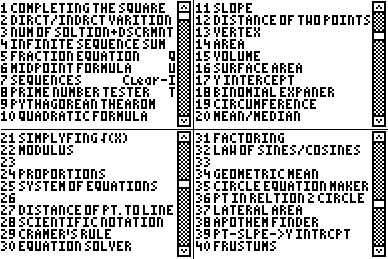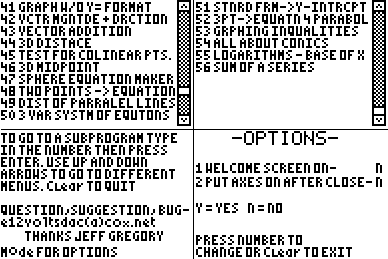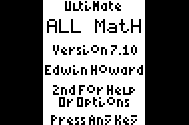Basics Archives Community Services ProgrammingHome :: Archives :: File Archives :: Allmath v7.14Allmath v7.14

FILE INFORMATION

allmath_84ce.zip
 Filename allmath_84ce.zip (Download) Title Allmath v7.14 Description Updated for TI-84 CE! Allmath is an all-inclusive math program that will calculate and do a variety of things (work and equations shown on most functions) including: complete the square, direct/indirect variation, number of solutions, midpoint, prime numbers, Pythagorean theorem, quadratic formula, slope, distance, vertex, area, volume, surface area, y intercept, Fibonacci sequence, circumference, average, simplified square roots, proportions, system of equations, distance of point to line, types of quadrilaterals, scientific notation, a point of an isosceles trapezoid, Pythagorean triples, law of sines/cosines, DMS, geometric mean, circle equation maker, lateral area, apothem finder, point-slope to Y-intercept, frustums, graphing without Y= format, graphing < and >, vector magnitude and direction, vector addition, and 3D distance, test for collinear points, 3D midpoint, sphere equation maker, distance of parallel lines, modulus, 3 variable system of equations, standard form to y intercept, cramers rule, 3 points to equation for parabola, graphing inequalities, All about conics (equation makers, details about, and etc.), logarithms of X base, iterations, sequences, sum of sequences, Cramer's Rule for a system of 3 equations. Allmath is compatible with MirageOS. This version is specific to TI-84+ C/CE calculators, for TI-83/84 calculators see corresponding version on ticalc directory. Recommended for Pre-algebra through Calculus. Version 7.14. Author Edwin Howard (e12voltsdac@gmail.com) Category TI-84 Plus C Silver Edition/CE BASIC Math Programs File Size 11,674 bytes File Date and Time Sun May 1 16:37:02 2022 Documentation Included? Yes

SCREEN SHOTSREVIEWS

There are no reviews for this file.

LEAVE FEEDBACK

Questions, comments, and problems regarding the file itself should be sent directly to the author(s) listed above.

Report inappropriate or miscategorized file (requires an account; or you may email us directly)